Ex 6.4

Chapter 6 Class 8 Squares and Square Roots
Serial order wise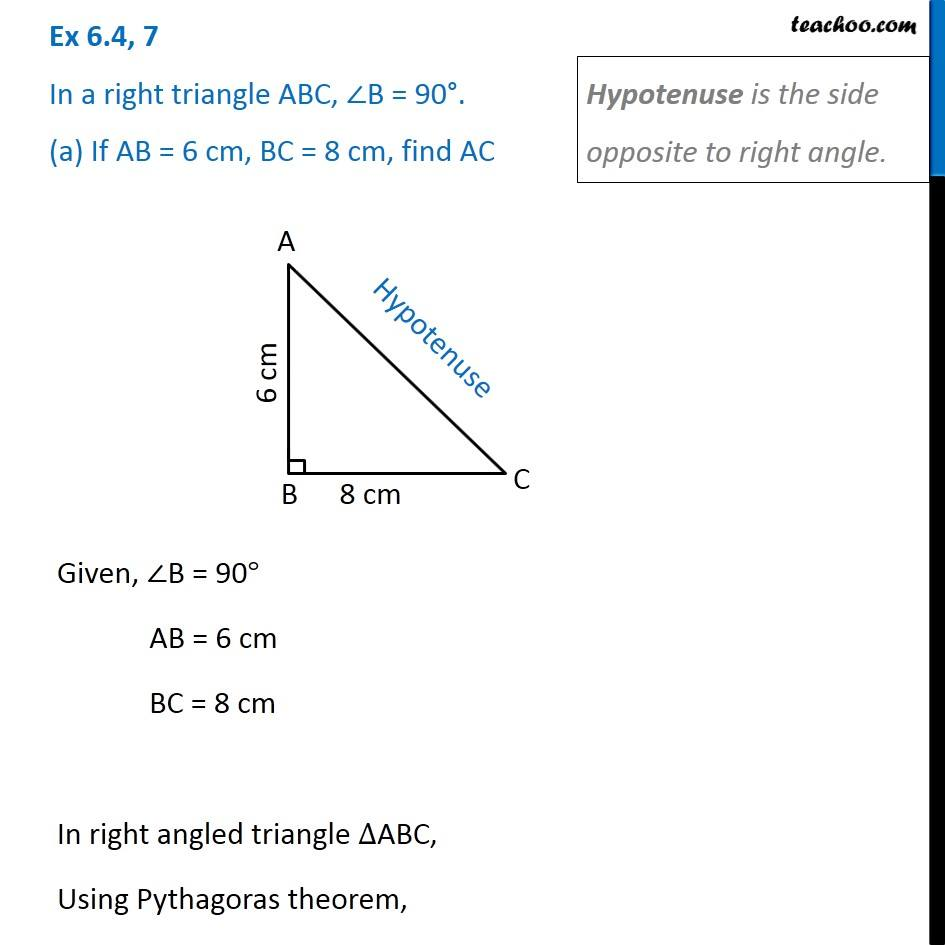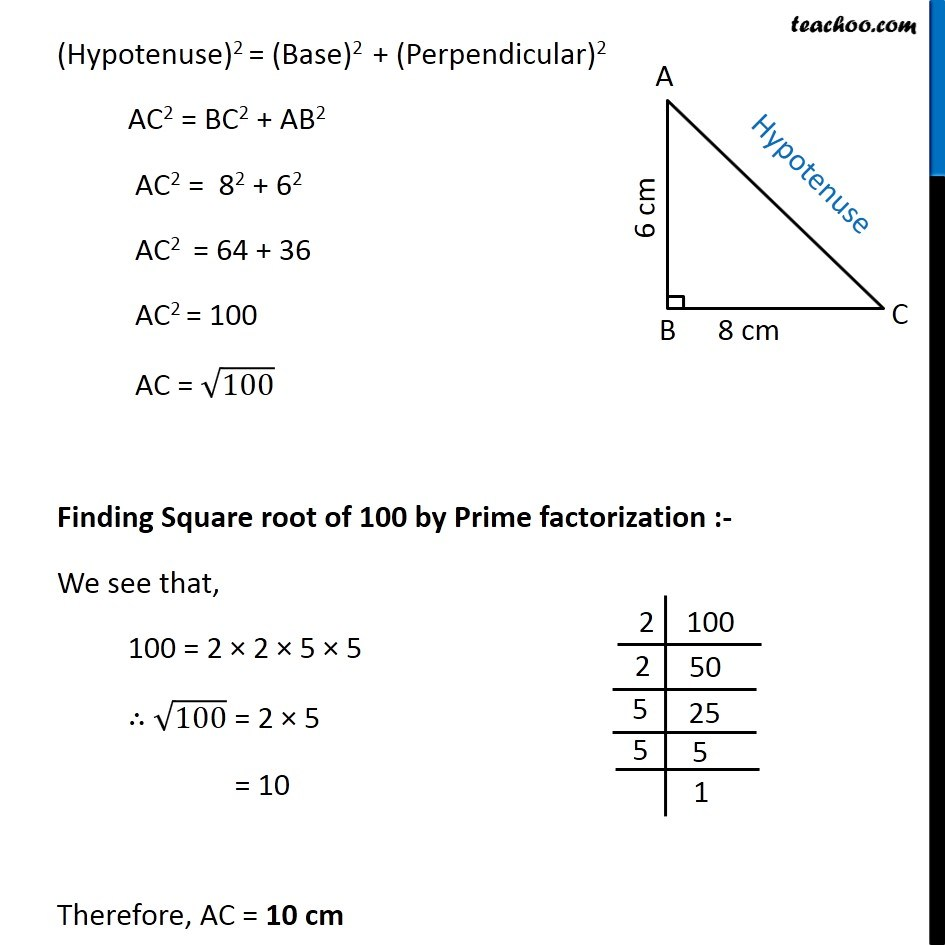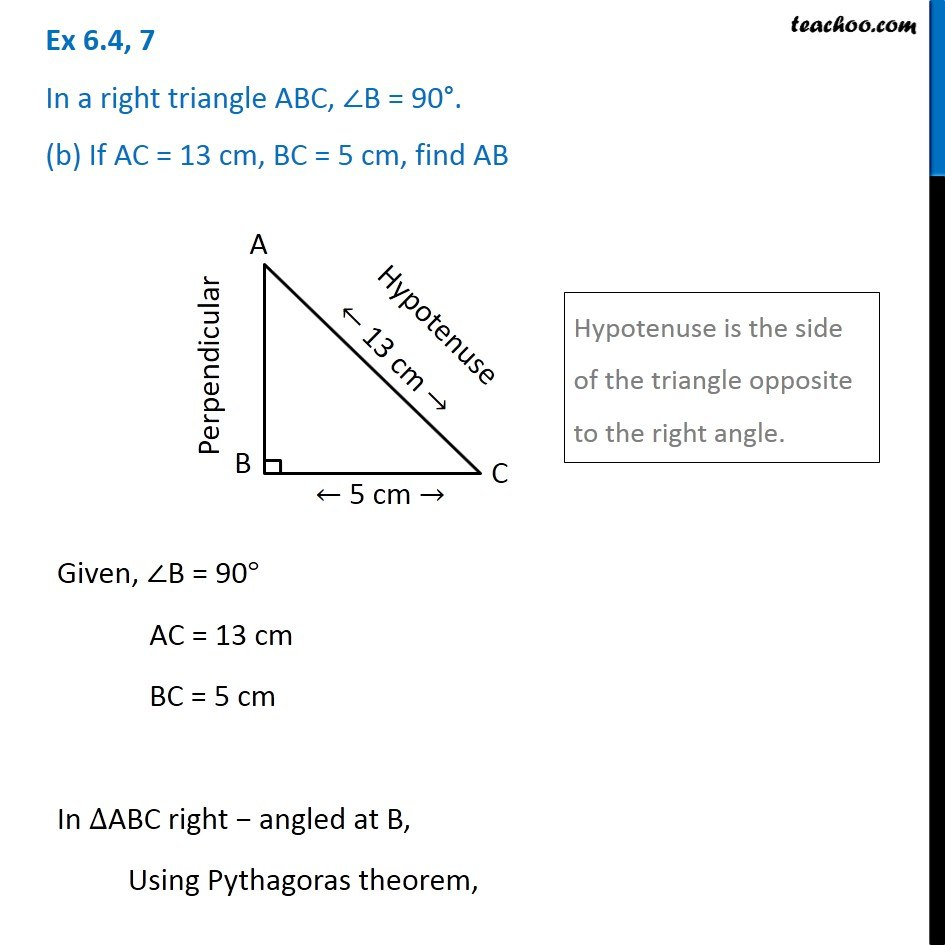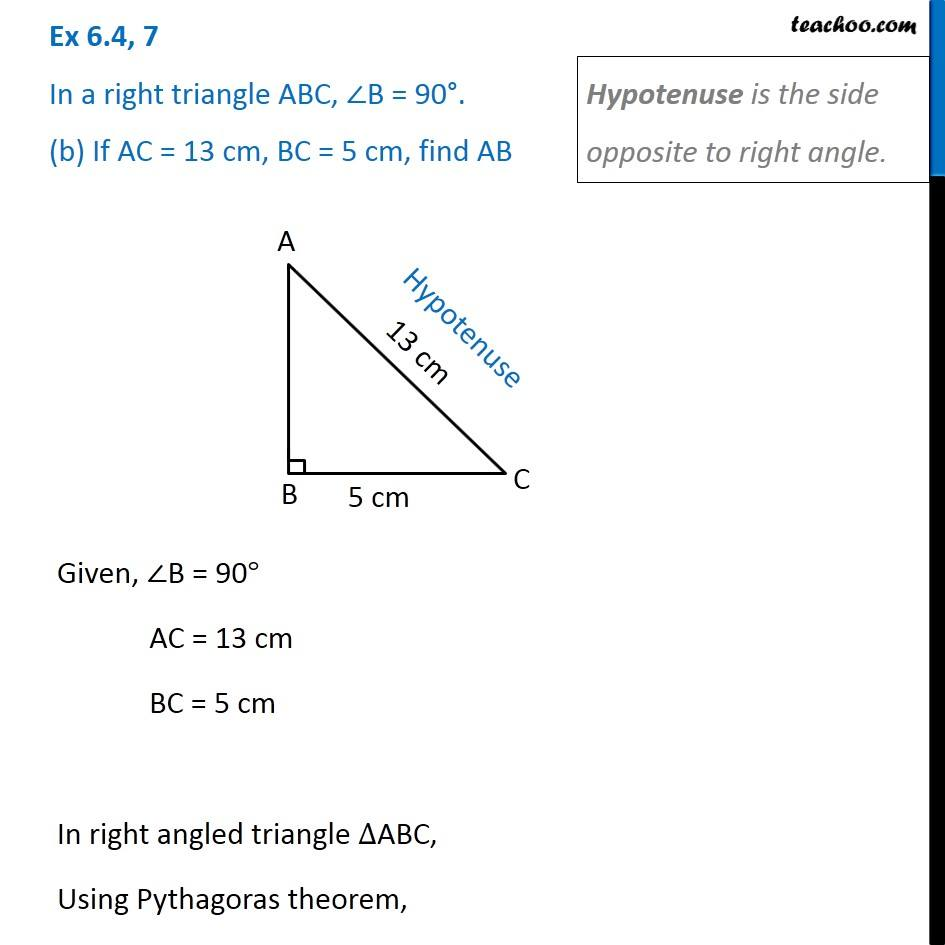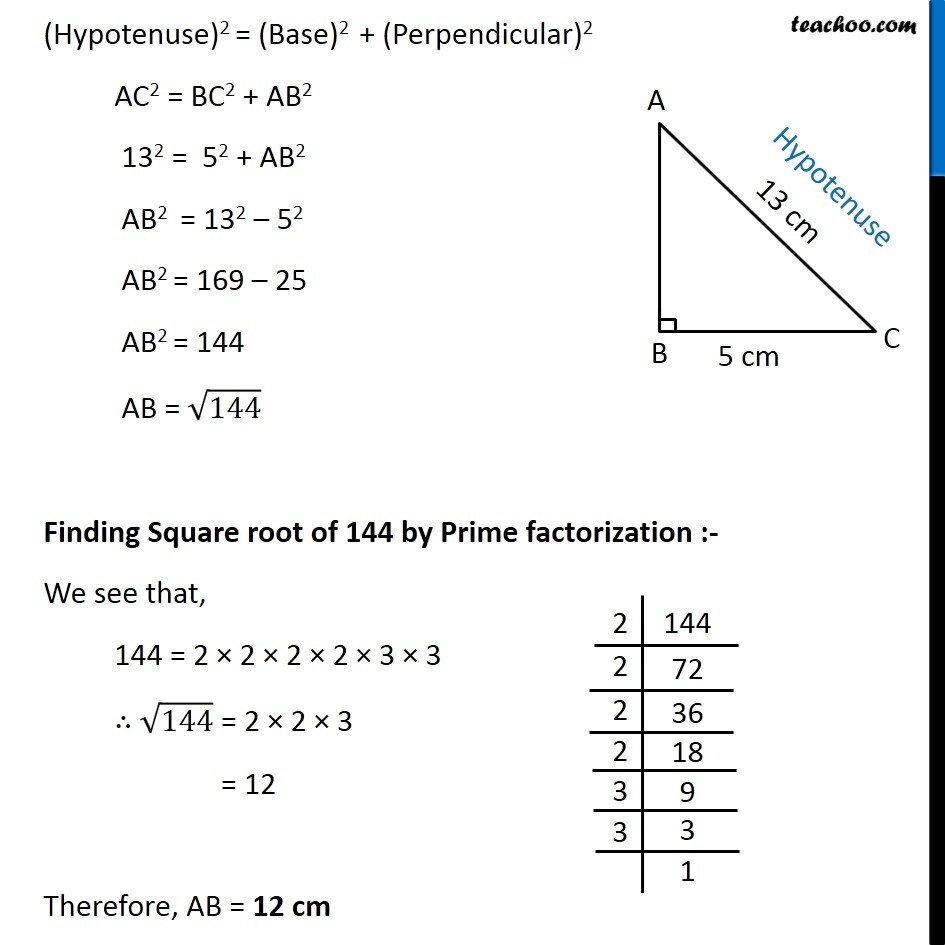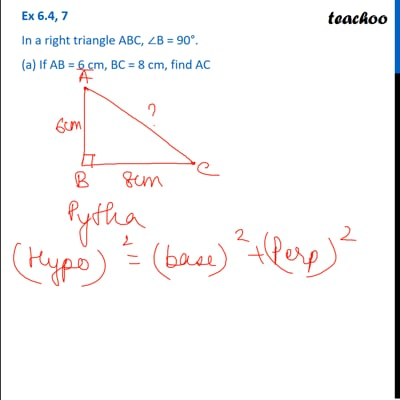This video is only available for Teachoo black users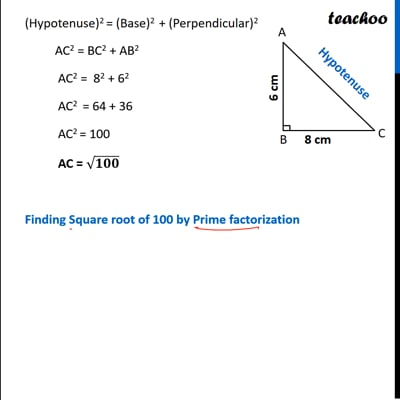This video is only available for Teachoo black users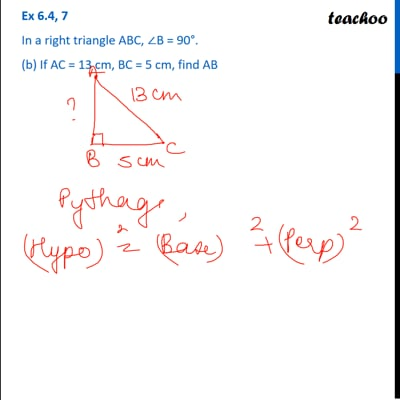This video is only available for Teachoo black users

Get live Maths 1-on-1 Classs - Class 6 to 12

### Transcript

Ex 6.4, 7 In a right triangle ABC, ∠B = 90°. (a) If AB = 6 cm, BC = 8 cm, find ACHypotenuse is the side opposite to right angle. Given, ∠B = 90° AB = 6 cm BC = 8 cm In right angled triangle ∆ABC, Using Pythagoras theorem, (Hypotenuse)2 = (Base)2 + (Perpendicular)2 AC2 = BC2 + AB2 AC2 = 82 + 62 AC2 = 64 + 36 AC2 = 100 AC = √100 Finding Square root of 100 by Prime factorization :- We see that, 100 = 2 × 2 × 5 × 5 ∴ √100 = 2 × 5 = 10 Therefore, AC = 10 cm Ex 6.4, 7 In a right triangle ABC, ∠B = 90°. (b) If AC = 13 cm, BC = 5 cm, find ABHypotenuse is the side of the triangle opposite to the right angle. Given, ∠B = 90° AC = 13 cm BC = 5 cm In ∆ABC right − angled at B, Using Pythagoras theorem, Ex 6.4, 7 In a right triangle ABC, ∠B = 90°. (b) If AC = 13 cm, BC = 5 cm, find ABHypotenuse is the side opposite to right angle. Given, ∠B = 90° AC = 13 cm BC = 5 cm In right angled triangle ∆ABC, Using Pythagoras theorem, (Hypotenuse)2 = (Base)2 + (Perpendicular)2 AC2 = BC2 + AB2 132 = 52 + AB2 AB2 = 132 – 52 AB2 = 169 – 25 AB2 = 144 AB = √144 Finding Square root of 144 by Prime factorization :- We see that, 144 = 2 × 2 × 2 × 2 × 3 × 3 ∴ √144 = 2 × 2 × 3 = 12 Therefore, AB = 12 cm